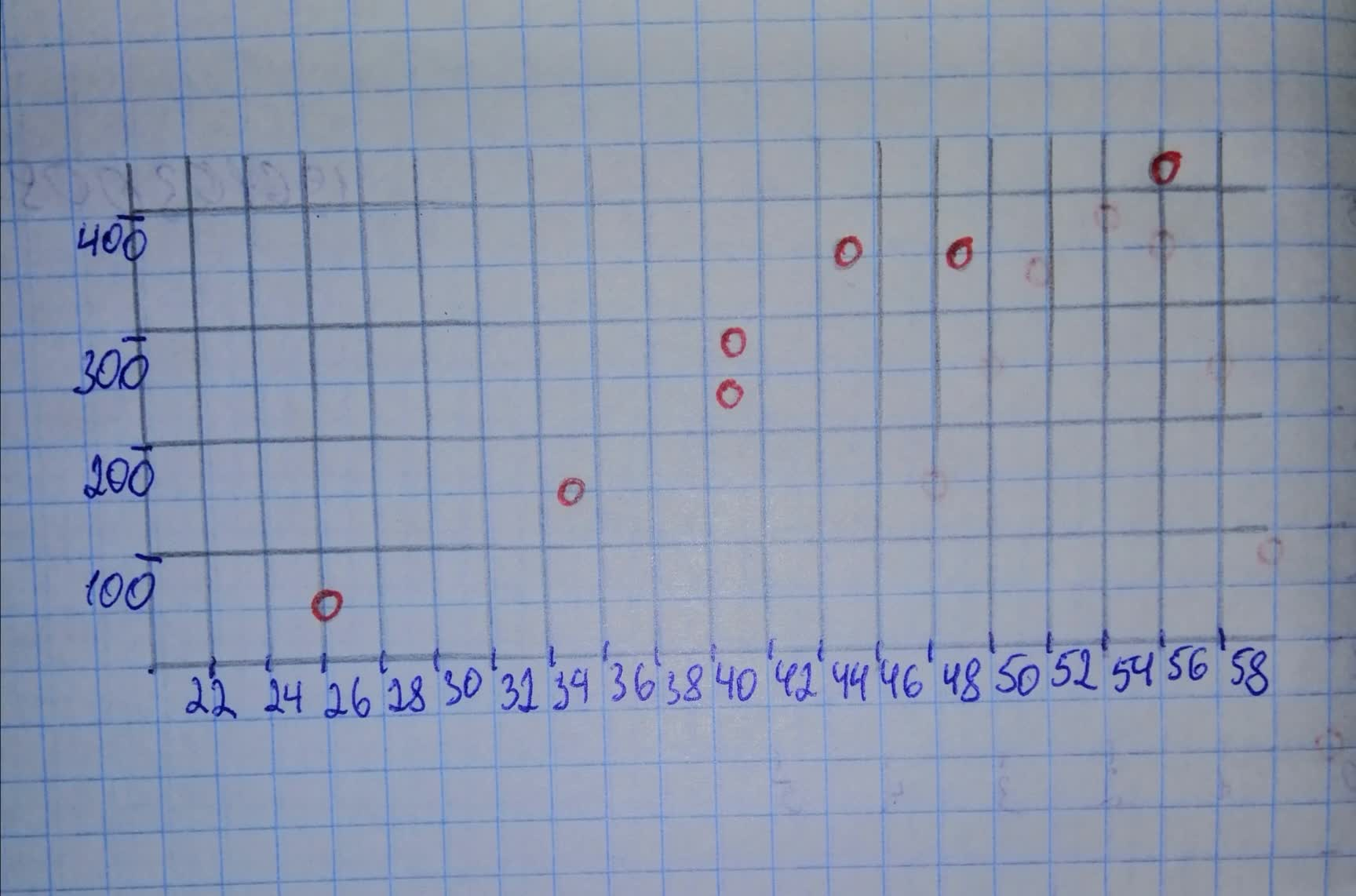# Use the sample data to construct a scatterplot.Use the first variable for the x-axis. Based on the scatterplot, what do you concluderingearV 2020-12-05 Answered

Use the sample data to construct a scatterplot.
Use the first variable for the x-axis. Based on the scatterplot, what do you conclude about a linear correlation?
The table li sts che t sizes (di stance around chest in inches) and weights (pounds) of anesthetized bears that were measured.
$\begin{array}{|cccccccc|}\hline \text{Chest(in.)}& 26& 45& 54& 49& 35& 41& 41\\ \text{Weight(lb)}& 80& 344& 416& 348& 166& 220& 262\\ \hline\end{array}$

You can still ask an expert for help

• Questions are typically answered in as fast as 30 minutes

Solve your problem for the price of one coffee

• Math expert for every subject
• Pay only if we can solve itArham Warner
Step 1
SCATTERPLOT
Chest is on the horizontal axis and Weight is on the vertical axis.Step 2
The appears to be a linear correlation, because the points in the scatterplot lie roughly on a straight line.